Math Calculators, Lessons and Formulas

It is time to solve your math problem

mathportal.org
• Geometry
• Triangles
• Mixed problems

# Mixed problems

ans:
syntax error
C
DEL
ANS
±
(
)
÷
×
7
8
9
4
5
6
+
1
2
3
=
0
.
auto next question
calculator
•  Question 1: 1 pts $\dfrac{x}{16}=\sin 52^{\circ}$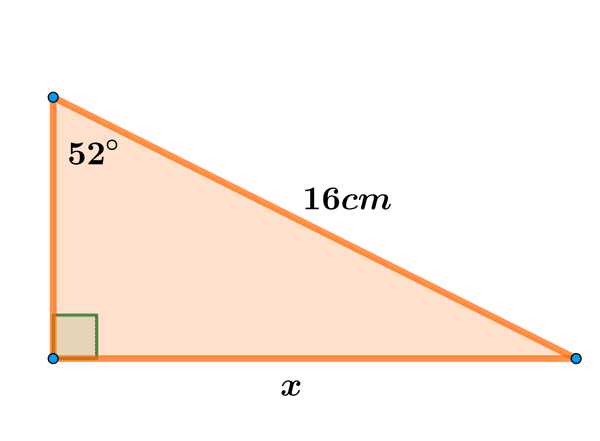•  Question 2: 1 pts $30cm, 40cm, 80cm$ can be the sides of a triangle.
•  Question 3: 1 pts Can $30^{\circ}, 40^{\circ}$ and $100^{\circ}$ be the angles of a triangle?
•  Question 4: 1 pts Find the area of an equilateral triangle that has sides equal to 6 cm.
 $A=6\sqrt{3}cm^{2}$ $A=9\sqrt{3}cm^{2}$ $A=36\sqrt{3}cm^{2}$ $A=72\sqrt{3}cm^{2}$
•  Question 5: 2 pts Find the ratio of the sides of a triangle whose interior angles are $30^{\circ}, 60^{\circ}$ and $90^{\circ}.$
$a:b:c=$
•  Question 6: 2 pts Find the side length of an equilateral triangle whose altitude is $12$ inches.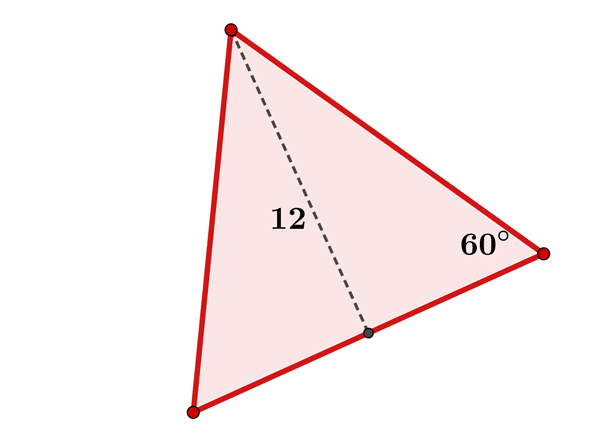•  Question 7: 2 pts Triangle $ABC$ shown below is inscribed inside a square of side 18 cm. Find the area of the triangle.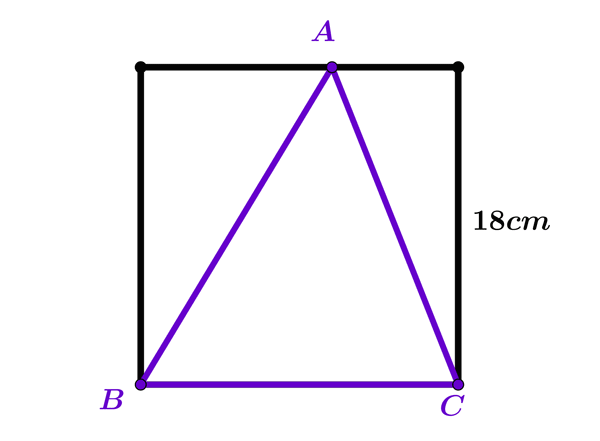$162cm^{2}$ $160cm^{2}$ $324cm^{2}$ $9cm^{2}$
•  Question 8: 2 pts The right triangle�shown below has an area of $25.$ Find its hypotenuse.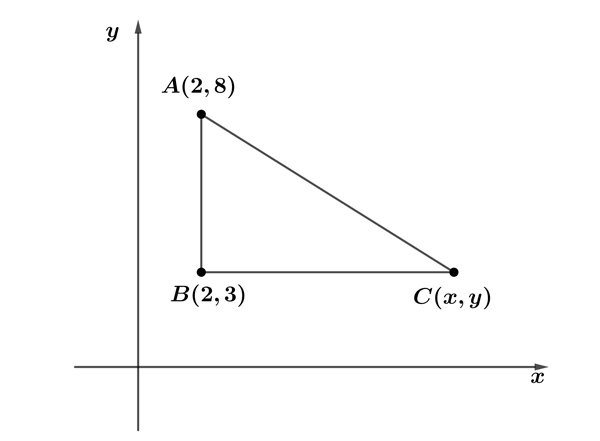$5$ $\sqrt{5}$ $5 \sqrt{5}$ $\dfrac{1}{5}$
•  Question 9: 3 pts Area of a triangle is $5m^{2},$ the length of one side is $4m$ and of other side $3m.$ Find the sine of the angle between given sides.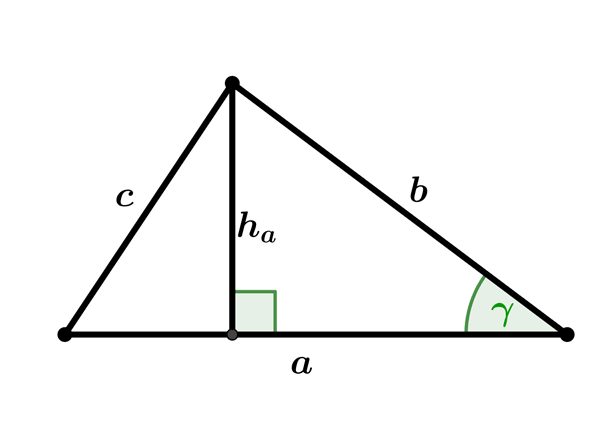$\sin \gamma =$
•  Question 10: 3 pts Angles of a triangle $\triangle ABC,$ which lay at the side $AB$ are $70^{\circ}$ and $80^{\circ},$ and $O$ is the intersection of altitudes of the triangle and $\delta=\measuredangle AOB.$ Find $\sin \delta.$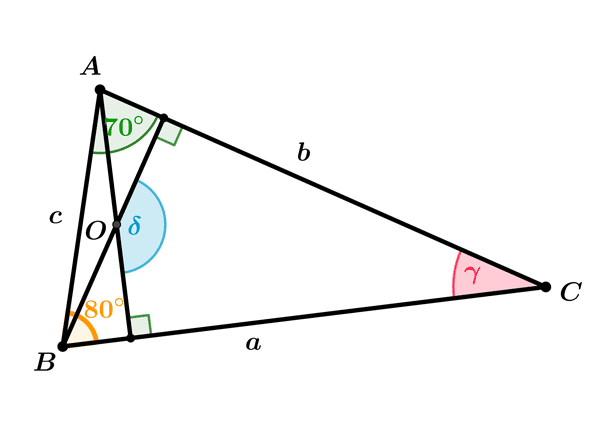$\sin \delta =$
•  Question 11: 3 pts Triangle $ABC$ is an isosceles triangle. The length of the base is $20$ meters and the corresponding height is $24$ meters. Find the perimeter of $ABC.$ (round your answer to the nearest tenth of a meter).
 $O=144m$ $O=72m$ $O=76m$ $O=18m$
•  Question 12: 3 pts The perimeter of a triangle is $74$ inches. The length of the first side is twice the length of the second side. The third side is $4$ inches more than the first side. Find the length of each side of the triangle.�